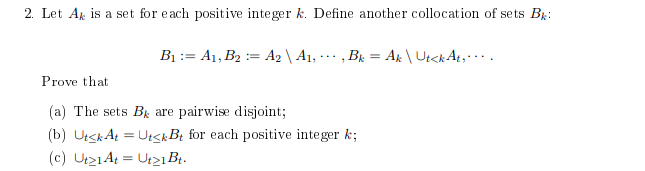# Thread: Need a bit of help with indexed sets

1. ## Need a bit of help with indexed sets

Hi,

I'm a real dunce when it comes to indexed sets/Collections etc. I can't seem to understand this question at all, so I would really appreciate some help with the following question.

Let Ak is a set for each positive integer k. Define another collection of sets Bk:
B1 := A1, B2 := A2 \ A1, · · · , Bk = Ak \ Ut<kAt
, · · · .
Prove that
(a) The sets Bk are pairwise disjoint;
(b) Ut≤kAt = Ut≤kBt for each positive integer k;
(c) Ut≥1At = Ut≥1Bt
.

2. ## Re: Need a bit of help with indexed sets

I'm sorry this notation is just too cryptic.

what are Ut, kAt, and kBt?

3. ## Re: Need a bit of help with indexed sets

I'm sorry, I should have made it more clear.

Here's the image of the question:4. ## Re: Need a bit of help with indexed setsOriginally Posted by BlackZeppelinHi,I'm a real dunce when it comes to indexed sets/Collections etc. I can't seem to understand this question at all, so I would really appreciate some help with the following question.
Let Ak is a set for each positive integer k. Define another collection of sets Bk:
B1 := A1, B2 := A2 \ A1, · · · , Bk = Ak \ Ut<kAt
, · · · .
Prove that
(a) The sets Bk are pairwise disjoint;
(b) Ut≤kAt = Ut≤kBt for each positive integer k;
(c) Ut≥1At = Ut≥1Bt.
Suppose that $\{j,k\}\subset\mathbb{Z}^+~\&~j<k$
By definition ${B_k} = {A_k}\backslash \bigcup\limits_{t = 1}^{k - 1} {{A_t}}$
Suppose that $y\in B_j\cap B_k$ so that means $y\in A_j~\&~y\in A_k$.
Study the definition of $A_k$ carefully. Has not $Aj\cap A_k$ been removed from $A_k$(recall $j<k$) to get $B_k~?$
Is that a contradiction?

5. ## Re: Need a bit of help with indexed setsOriginally Posted by PlatoSuppose that $\{j,k\}\subset\mathbb{Z}^+~\&~j<k$
By definition ${B_k} = {A_k}\backslash \bigcup\limits_{t = 1}^{k - 1} {{A_t}}$
Suppose that $y\in B_j\cap B_k$ so that means $y\in A_j~\&~y\in A_k$.
Study the definition of $A_k$ carefully. Has not $Aj\cap A_k$ been removed from $A_k$(recall $j<k$) to get $B_k~?$
Is that a contradiction?
If I'm correct, then yes it is a contradiction. Since Bk is supposed to have no element from any set denoted by an integer less than k. That proves that the sets are pairwise disjoint. So, that's a bit clear now, thanks!

But what do parts b) and c) even imply. I don't even understand their meaning...

6. ## Re: Need a bit of help with indexed setsOriginally Posted by BlackZeppelinBut what do parts b) and c) even imply. I don't even understand their meaning...
Here are parts b) & c).

b) $\left( {\forall k} \right)\left[ {\bigcup\limits_{t = 1}^k {{A_t}} = \bigcup\limits_{t = 1}^k {{B_t}} } \right]$

c) $\bigcup\limits_{t = 1}^\infty {{A_t}} = \bigcup\limits_{t = 1}^\infty {{B_t}}$

See what you can do with those.

7. ## Re: Need a bit of help with indexed sets

I know it is late in Lahore. Here are some clues.
Can you see that $(\forall k)[B_k\subset A_k]$ If so that is the trick for b).

Say $x\in\bigcup\limits_{t = 1}^\infty {{A_t}}$ then $(\exists j)[x\in A_j]$
Let $n$ be the least such $j$. If $n=1$ then $A_n=B_1$ and we are done.

But if $n>1$ then $x\notin A_k$ for $k=1,\cdots n-1$ which means that $x \in {B_n} = {A_n}\backslash \bigcup\limits_{t = 1}^{n - 1} {{A_t}}$

The other direction. Suppose that $y\in\bigcup\limits_{t = 1}^\infty {{B_t}}$ Can you finish?